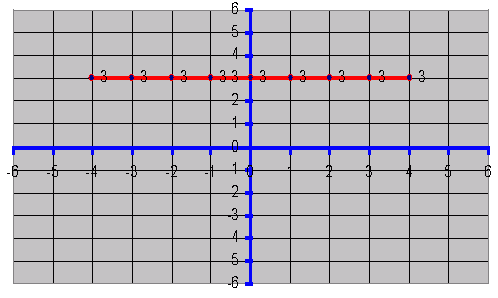Name: ___________________Date:___________________

 Plot the following point on a graph sheet (2,1),(2,2),(2,3),(2,4),(2,5),(2,0),(2,-1),(2,-2),(2,-3),(2,-4) and (2,-5).Observing the above graph: 1. All these points lie on a straight line which is perpendicular to X-axis. 2. All these points lie on a straight line which is parallel to Y-axis. 3. The x-coordinate is constant for all these points.Ā That is x = 2. 4. The common property of all these points on the straight line is x = 2. 5. x = 2 is a straight line parallel to Y-axis and at a distance of 2 units right and it intersects the X-axis at the point (2,0). x = 2 is a relation and this is satisfied by all those ordered pairs in which the first coordinate x is always 2 and the second coordinate y is any real number. Draw the graph of y = 3. All the points whose second coordinate y is 3 and whose first coordinate is any real number satisfy this relation.Ā Some ordered pairs that satisfy the given relation are (0,3),(1,3),(3,3),(4,3),(-1,3),(-2,3),(-3,3) and (-4,3).Observing above graph, all these ordered pairs when plotted lie on a straight line which is parallel to the X-axis.Ā The straight line y=3 is parallel to X-axis and it intersects Y-axis at the point (0,3). Directions: Draw the graph for linear equations. Verify using TI 83 or TI 84 graphing calculator. x = 1 x = 2 x = 3 x = 4 y = 1 y = 2 y = 3 y = 4Name: ___________________Date:___________________